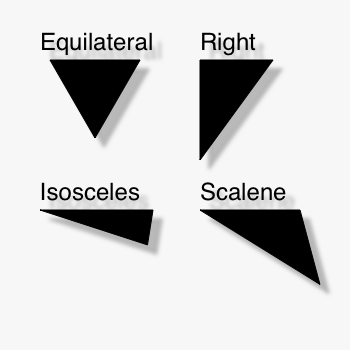# Triangles

Posted by ideambulate on Jun 07, 2008I've implemented a simple set of self-contained functions for producing the classic varieties of triangles: equilateral, right, isosceles, and scalene. They return path objects, so you can play with the outputs handily.

Naturally, there are other ways of parameterizing triangles than the input arguments I've chosen, but these seemed the easiest for me to visualize. The "coordinates" function is included in all of them, just for the sake of modularity.

```def equilateraltriangle(x0,y0,length,draw=True):
'''Draws an equilateral triangle.'''
def coordinates(x0, y0, distance, angle):
from math import radians, sin, cos
x = x0 + cos(radians(angle)) * distance
y = y0 + sin(radians(angle)) * distance
return x, y

x1,y1 = coordinates(x0,y0,length,0)
x2,y2 = coordinates(x0,y0,length,60)
beginpath(x0,y0)
lineto(x1,y1)
lineto(x2,y2)
lineto(x0,y0)
p = endpath(draw)
return p

def righttriangle(x0,y0,length1,length2,draw=True):
'''Draws a right triangle, with the right angle at the origin'''
def coordinates(x0, y0, distance, angle):
from math import radians, sin, cos
x = x0 + cos(radians(angle)) * distance
y = y0 + sin(radians(angle)) * distance
return x, y

x1,y1 = coordinates(x0,y0,length1,0)
x2,y2 = coordinates(x0,y0,length2,90)
beginpath(x0,y0)
lineto(x1,y1)
lineto(x2,y2)
lineto(x0,y0)
p = endpath(draw)
return p

def isoscelestriangle(x0,y0,length,angle,draw=True):
'''Draws an isosceles triangle defined by the length of the
two equal sides and the angle between them.'''
def coordinates(x0, y0, distance, angle):
from math import radians, sin, cos
x = x0 + cos(radians(angle)) * distance
y = y0 + sin(radians(angle)) * distance
return x, y
a = 180 - 2*angle
x1,y1 = coordinates(x0,y0,length,0)
x2,y2 = coordinates(x0,y0,length,a)
beginpath(x0,y0)
lineto(x1,y1)
lineto(x2,y2)
lineto(x0,y0)
p = endpath(draw)
return p

def scalenetriangle(x0,y0,length1,length2,angle,draw=True):
'''Draws a scalene triange defined by the length of two sides
and the angle between them.'''
from math import radians, sin, cos,asin,degrees
def coordinates(x0, y0, distance, angle):
from math import radians, sin, cos
x = x0 + cos(radians(angle)) * distance
y = y0 + sin(radians(angle)) * distance
return x, y

a,c,angB = length1,length2,angle

x1,y1 = coordinates(x0,y0,a,0)
x2,y2 = coordinates(x0,y0,b,angC)

beginpath(x0,y0)
lineto(x1,y1)
lineto(x2,y2)
lineto(x0,y0)
p = endpath(draw)
return p```

Posted by ideambulate on Jun 10, 2008

Pretty trivial, I know, but maybe it'll save someone out there a bit of geometric review.

Posted by Tom De Smedt on Jun 27, 2008

Hi ideambulate,

Thanks for sharing your code. We've also had previous posts for spirals and polygons. In the future it will become much easier to extend NodeBox with this kind of functionality, e.g. NodeBox would have an online, community-driven "node-repository" from which you can pick nodes and customize your own NodeBox.

This is part of the stuff we're working on right now, and the reason why it has been a little bit quiet on the forum lately. You can probably expect some first results around the start of 2009.